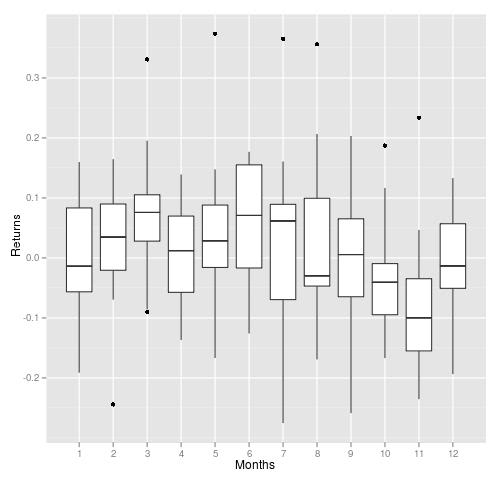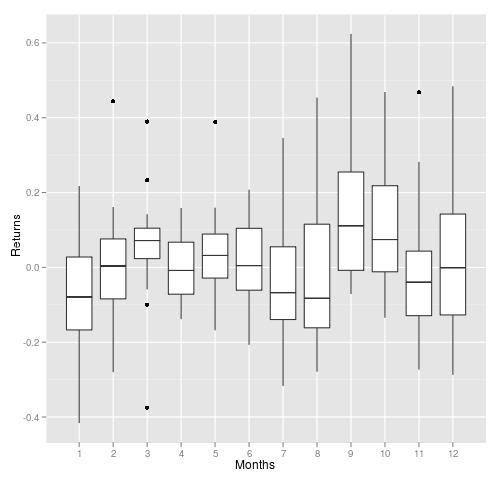# Gas price seasonality

February 18, 2010
By

Want to share your content on R-bloggers? click here if you have a blog, or here if you don't.

Last spring I read “Quantitative Trading” by Ernest P. Chan. In his book, he suggested to buy gas futures contract at the end of February and sell it later, in March. Today, I decided to test this strategy by using R-language.
The most important thing for such investigation is data. For this purpose, I used this public data: www.eia.doe.gov
I made 2 test – for the first one, I used futures contract, which will be settled after 4 months and for the second one, I used gas spot price.
In the first plot, we can see monthly price returns scaled by months (1-12). It is clear, that mean of March’s returns are above 0. What is encouraging in this plot is that the ranges of returns are above 0 as well (meaning, that majority of March’s returns was above 0).
Anova test gives p-value below 0.01 – some months in the group has different mean (that supports seasonality idea). March’s t-test gives these values: t = 2.8064, df = 15, p-value = 0.01329.I used gas spot prices to generate second plot. It is similar to the first one, except that March’s returns has longer tail. It is worth to note, that September stands as a positive month as well. The statistics for this test are not so strong, as it was in the first example.
P-value of Anova test is only 0.11 and March’s t-test is:
t = 1.3791, df = 15, p-value = 0.1881R-language code to run these tests:

``` #------gas contract 4 ----- library(xts) library(ggplot2) library(quantmod)   tmp<-as.matrix(read.table(file='gas.contract.4.csv',sep=';')) gas<-as.xts(as.double(tmp[,2]),order.by=as.POSIXct(tmp[,1])) plot(gas) gas.monthly<-to.monthly(gas) gas.monthly.delta<-Delt(Cl(gas.monthly)) gas.monthly.delta<-0   gas.factor<-as.double(format(index(gas.monthly.delta),'%m')) tmp<-data.frame(as.double(gas.monthly.delta),as.numeric(gas.factor)) qplot(factor(as.numeric(gas.factor)),as.double(gas.monthly.delta),data=tmp,geom = "boxplot",ylab='Returns',xlab='Months')   anova(lm(as.double(gas.monthly.delta)~gas.factor)) t.test(((gas.monthly.delta[which(format(index(gas.monthly.delta),'%m')=='03')]))[,1])   #----gas contract spot ------- tmp<-as.matrix(read.table(file='gas.csv',sep=';')) gas<-as.xts(as.double(tmp[,2]),order.by=as.POSIXct(tmp[,1]))   plot(gas) colnames(gas)<-c('Close')   gas.monthly<-to.monthly(gas) gas.monthly.delta<-Delt(Cl(gas.monthly)) gas.monthly.delta<-0   gas.factor<-as.double(format(index(gas.monthly.delta),'%m')) tmp<-data.frame(as.double(gas.monthly.delta),as.numeric(gas.factor))   qplot(factor(as.numeric(gas.factor)),as.double(gas.monthly.delta),data=tmp,geom = "boxplot",ylab='Returns',xlab='Months')   anova(lm(as.double(gas.monthly.delta)~gas.factor)) t.test(((gas.monthly.delta[which(format(index(gas.monthly.delta),'%m')=='03')]))[,1]) ```

R-bloggers.com offers daily e-mail updates about R news and tutorials about learning R and many other topics. Click here if you're looking to post or find an R/data-science job.
Want to share your content on R-bloggers? click here if you have a blog, or here if you don't.

Tags: , ,